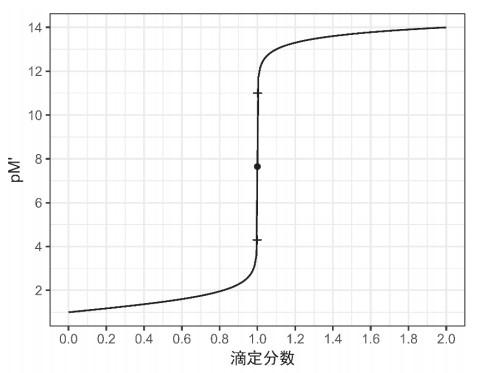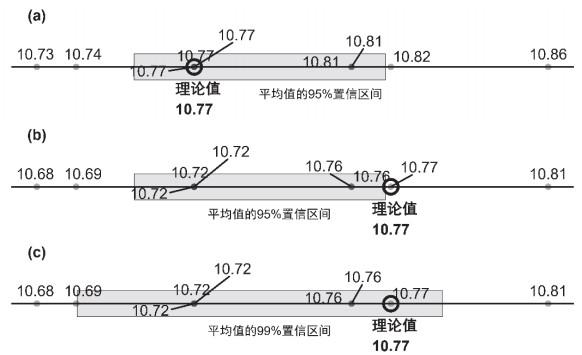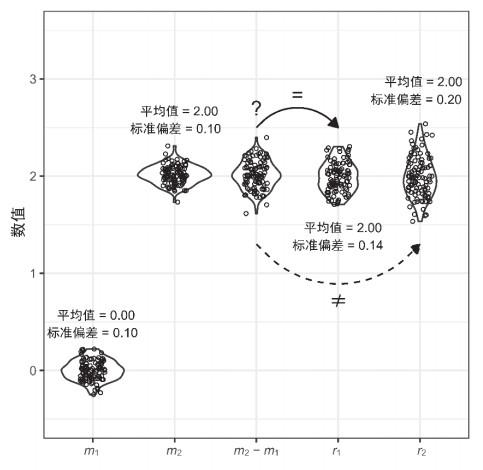## Application of Programming Language R in Teaching of Analytical ChemistryAbstract

Application of programming language R for the teaching of analytical chemistry was demonstrated with several examples. Numerical calculation of R was used to solve the high-order equations involved in chemical equilibrium. It avoided the cumbersome processes of formula simplification, and also unified all equilibrium calculations into the same method. Plotting functions of R were used to plot species distribution curves, titration curves, etc. Statistical analysis functions of R were used to replace the traditional manual method. Numerical simulation functions of R were used to help students understand complex concepts such as propagation of error and confidence interval. Compared with Excel, which is commonly used in the teaching of analytical chemistry, R also has the characteristics of being code-based, self-explanatory, flexible, rapidly evolving, and readily accessible. The application of R in teaching of analytical chemistry not only helps to improve the learning efficacy, but also provides an opportunity for students to master the powerful research tool R.

Keywords： Analytical chemistry ; R programming language ; pH calculation ; Species distribution ; Titration curve ; t test ; Propagation of error

Tan Qiaoguo. Application of Programming Language R in Teaching of Analytical Chemistry. University Chemistry[J], 2021, 36(2): 2005007-0 doi:10.3866/PKU.DXHX202005007

R语言是近年来兴起的一门计算机语言，在生物学、生态环境科学、经济学、社会科学等各领域都得到了广泛的应用，成为当前最受欢迎的编程语言之一[9, 10]。R有比Excel更卓越的数据处理和可视化能力，理论上非常适用于分析化学的教学。然而，与其在科学研究中的热度形成鲜明对比，目前尚未见论文和书籍介绍将R用于包括分析化学在内的课程教学。本文列举数例，说明R可以在分析化学教学的多个方面得到应用。

## 1 R语言简介

R语言是一款开源免费的编程语言，主要用于统计分析和数据可视化。R最初是由两位统计学家罗斯∙伊哈卡和罗伯特∙杰特曼基于S语言开发而成。由于两位开发者名字的首字母都是R，R因此得名。目前R由“R开发核心团队”负责维护和开发

R的功能可以通过程序包得到扩展。例如本文中绘图用到了ggplot2程序包，数据整理用到了reshape2程序包，而这两个程序包与其他多个常用的程序包一起又包括在tidyverse程序包中。初次使用时，需运行以下代码安装：

install.packages("tidyverse")

R以代码形式实现计算和作图，操作过程完整地体现在代码中。代码具有自释性，理论上无需另外的解释性文字。代码可以纯文本的形式储存和传播，操作过程能够通过代码精确重现。与此相比，Excel计算过程的展示则必须用到图片(甚至视频)结合繁琐的步骤说明，不仅占用大量储存空间，而且也不利于教学中的传播、分享和交流。

R有广阔的应用场景。R作为以数据分析和可视化见长的编程语言，是科学研究的有力工具。R借助扩展程序包，不断发展出新的功能满足科研需求。在分析化学的教学中使用R，为学习者接触掌握这项科研利器提供良好契机，可提高学习者的知识获得感和实际收益。

R软件免费，轻便。在写作本文时，最新版本R 4.0.0的安装文件仅84 MB。因此便于在不同的场合(如教室、师生的个人电脑等)随时安装使用R。与此相比，Matlab等收费软件不仅价格昂贵，而且需要5–8 GB的安装空间。

### 2.1 R应用于分析化学计算

R语言可看成一个功能全面的计算器，能执行各类科学计算，能用于解决分析化学中的高次方程求解问题。下面举一例说明求解过程。例题取自柳青和王海水的论文，关于磷酸溶液pH值的计算。柳青和王海水提出了简化多元弱酸溶液pH值计算的新方法，但思路仍然是将高次方程简化为二次或一次方程。其方法改善了通用性，但过程仍然较为复杂，仍有较大的记忆负担。

#输入已知条件

Ka1 = 7.6E-3

Ka2 = 6.3E-8

Ka3 = 4.4E-13

c = 0.20

#定义三元酸H+浓度的精确计算式

f = function(H) {

#将分母命名为D

D = H^3 + H^2*Ka1 + H*Ka1*Ka2 + Ka1*Ka2*Ka3

H - 1E-14/H - (c*H^2*Ka1 + c*2*H*Ka1*Ka2 + c*3*Ka1*Ka2*Ka3)/D

}

#运用uniroot函数解方程

H = uniroot(f, interval = c(0, 0.6), tol = 10^-20)$root #根据H+浓度计算 pH = -log10(H) pH 以上代码中，起核心作用的是解方程函数uniroot()。在使用uniroot()时，所输入的f是H+浓度精确计算式，因溶液体系而异；参数interval是粗略预估的方程根的上下限；参数tol是所允许的误差。运行以上代码后，得到结果：1.45134，因此可知溶液pH值为1.45，与参考文献中的计算值一致。 运用该方法，除了计算准确，还可以将所有酸碱溶液的pH值计算都转变为同一种方法。计算过程可分为三步：(1)根据质子得失平衡写出质子条件式；(2)将式中酸(碱)各型体的浓度都替换成酸(碱)的分析浓度与分布分数之积，得到关于[H+]([OH])的一元高次方程，即以上所用的f；(3)解高次方程。 此方法只用到质子条件式和分布分数这两个容易掌握的基本方法，避免了复杂的判别式和简化过程，便于理解和记忆。从而使学习者从复杂的公式和繁琐的计算中解脱出来，将更多精力用于学习分析化学知识本身。 #### 2.2 R应用于分析化学绘图 R语言另一项特色功能是科学绘图。图在分析化学的教学中发挥着不可或缺的作用，可以将复杂的概念、溶液体系、反应过程等可视化，降低理解难度。例如，分布分数图之于化学平衡，滴定曲线图之于滴定原理，都是极为有效的教学工具。学习者若能自己绘制这些图，则更能提升学习效果，增加学习乐趣。 以下以多元酸的分布分数图和络合滴定图为例，演示如何用将R应用于分析化学绘图，并帮助理解其背后的化学原理。需要说明的是，为了节省篇幅，以下作图的R代码仅保留主干部分，图的细节修饰所需代码未给出。读者运行以下代码，可看到图的主体信息，但格式细节与文中所示的图略有区别。完整作图代码获取方式见文末说明。 #### 2.2.1 分布分数图的绘制 三元弱酸磷酸经三级解离，得到四种型体。根据磷酸的三个Ka值，可得到四种型体分布分数的表达式，并据此绘制分布分数图。以下是绘图的R代码： library(ggplot2) #加载用于绘图的程序包 Ka1 = 7.6E-3 Ka2 = 6.3E-8 Ka3 = 4.4E-13 #选取0~14范围内的100个pH值 pH = seq(from = 0, to = 14, length.out = 100) H = 10^(-pH) #根据H+浓度计算分布分数的分母 D = H^3 + H^2*Ka1 + H*Ka1*Ka2 + Ka1*Ka2*Ka3 #计算磷酸各型体的分布分数 delta_0 = Ka1*Ka2*Ka3/D delta_1 = H*Ka1*Ka2/D delta_2 = H^2*Ka1/D delta_3 = H^3/D #将pH值和各型体分布分数组合成数据表，用于绘图 df = data.frame(pH, delta_0, delta_1, delta_2, delta_3) #绘制分布分数图 ggplot(df, aes(x = pH))+ #x轴为pH geom_line(aes(y = delta_0), linetype = 1)+ #PO4对应的曲线 geom_line(aes(y = delta_1), linetype = 2)+ #HPO4对应的曲线 geom_line(aes(y = delta_2), linetype = 3)+ #H2PO4对应的曲线 geom_line(aes(y = delta_3), linetype = 4) #H3PO4对应的曲线 运行以上代码，可得到磷酸的分布分数图(图 1)。该图可用于判断不同pH值条件下，磷酸的主要型体。例如，天然海水的pH约为8.2，因此可判断海水中的磷酸盐主要以${\rm{HPO}}_4^{2 - }$型体存在。该图亦可反向用于估算磷酸或磷酸盐溶液的pH值。例如，可估算KH2PO4和K2HPO4溶液的pH值分别在5和10左右。例1中的问题亦可使用该图帮助简化。假设0.20 mol∙L−1的H3PO4溶于水后第一级电离发生了50% (此比例据经验粗略估算，无需准确)，则溶液pH值为1左右。据图 1判断，溶液中几乎不存在${\rm{HPO}}_4^{2 - }{\rm{PO}}_4^{3 - }$，因此可忽略H3PO4的第二、三级电离，可直接将磷酸当成一元弱酸来处理，大为简化了其pH值的计算。 ### 图1图1 用R绘制磷酸的分布分数图 除了一元、多元酸碱的分布分数图，其他分布分数图，如Cu(NH3)n逐级络合物的分布分数图，EDTA各型体的分布分数图等，亦可按照以上方法绘制。学习者若改变作图参数(如Ka值)，便可即时看到图的变化。这样的过程可以帮助理解稳定常数、解离常数等如何决定了曲线的形状，进而理解这些常数的内涵。 #### 2.2.2 滴定曲线的绘制 以下以EDTA络合滴定金属离子M为例，演示如何用R绘制络合滴定曲线。络合滴定曲线是滴定过程中金属离子浓度(pM')与滴定分数(a)之间关系的曲线，由络合滴定曲线方程描述：$ {K}_{\mathrm{M}\mathrm{Y}}^{\text{'}}·{\left[\mathrm{M}\mathrm{\text{'}}\right]}^{2}+[{K}_{\mathrm{M}\mathrm{Y}}^{\text{'}}·{c}_{\mathrm{M}}·\left(a-1\right)+1]·\left[\mathrm{M}\mathrm{\text{'}}\right]-{c}_{\mathrm{M}}=0 $在此例中，假设用0.1000 mol∙L−1的EDTA滴定同浓度的金属离子M，EDTA络合M的条件稳定常数K'MY为1.0 × 1014 L∙mol−1。绘制其滴定曲线图的R代码如下： #设定金属离子和EDTA溶液的分析浓度 cM = 0.1 #设定条件稳定常数值 KMY = 1E14 #设定滴定分数，在0~2之间取200个点 a_seq = seq(0, 2, length.out = 200) #设定名为pM，长度为200的向量，用于储存计算所得的pM值 pM = numeric(200) #针对每一个滴定分数值a，解络合滴定方程计算对应的pM值，此处运用循环语句 for (i in 1:200) { #定义络合滴定曲线方程 f = function(x) { #将已知条件导入方程 a < < - a_seq[i] KMY < < - KMY cM < < - cM KMY*x^2+(KMY*cM/(1+a)*(a-1)+1)*x-cM/(1+a) } #将方程的根存入名为M的变量 M = uniroot(f, interval = c(1E-20, 0.1), tol=1E-20)$root

#计算pM值并保存

pM[i] = -log10(M)

}

#将滴定分数值与对应的pM值合并成数据表，命名为df

df = data.frame(a = a_seq, pM = pM)

#调用作图所需软件包ggplot2

library(ggplot2)

#作图

ggplot(df, aes(x = a, y = pM))+

geom_line()+

labs(x = "滴定分数", y = "pM'")

### 图2·所示为化学计量点(滴定分数= 1.000)；+所示为滴定突跃范围(0.999 ≤滴定分数≤ 1.001)

#### 2.3 R应用于分析化学中的统计分析

#将9次测定值存入变量x

x = c(10.74, 10.77, 10.77, 10.77, 10.81, 10.82, 10.73, 10.86, 10.81)

#用t.test函数进行t检验，参数mu是理论值，参数conf.level是置信度

t.test(x, mu = 10.77, conf.level = 0.95)

##            One Sample t-test

## t = 1.2039, df = 8, p-value = 0.2631

## alternative hypothesis: true mean is not equal to 10.77

## 95 percent confidence interval:

## 10.75474 10.81859

### 图3(a) 95%置信区间包含理论值，无显著系统误差；(b) 95%置信区间不包含理论值，存在显著系统误差；(c) 99%置信区间比95%置信区间宽，包含理论值，无显著系统误差

library(ggplot2)

ggplot(data.frame(x), aes(x, y=0))+

geom_rect(xmin=10.75474, xmax=10.81859, ymin=-0.01, ymax=0.01)+ #置信区间

geom_point(alpha = 1/3)+ #各测定值对应的点

geom_text(label=x, nudge_y=0.01)+ #各测定值

geom_point(x=10.77, y=0, shape= 1, size =4)+ #理论值

ylim(-0.1, 0.1)

x_2 = x - 0.05 #原数据各减去0.05

t.test(x_2, mu = 10.77, conf.level = 0.95)

t.test(x_2, mu = 10.77, conf.level = 0.99)

#### 2.4 R数值模拟用于解释复杂概念

R作为编程语言，有生成符合特定分布的随机数，进行随机抽样等功能，因而可以进行数值模拟，帮助解释分析化学中一些复杂的概念和方法，以下举一例说明。

### 图4(m2m1)的标准偏差与m1m2的标准偏差的关系以及与标准偏差0.20和0.14的对比

library(ggplot2)

library(reshape2)

#随机生成4组包含100个符合正态分布的数，参数mean为均值，sd为标准偏差

m1 = rnorm(100, mean = 0, sd = 0.10) #均值为0，标准差为0.10

m2 = rnorm(100, mean = 2, sd = 0.10) #均值为2，标准差为0.10

r1 = rnorm(100, mean = 2, sd = 0.20) #均值为2，标准差为0.20

r2 = rnorm(100, mean = 2, sd = 0.1414) #均值为2，标准差为0.1414

#计算m2与m1之差

m = m2 - m1

#运用data.frame函数将上述5组数合组合成数据表d

d = data.frame(m1, m2, r1, r2, m)

#运用gather函数排列的数据表d的转化成纵向排列的数据表d_1

d1 < - melt(d)

#运用ggplot函数作图

ggplot(d1, aes(x = variable, y = value))+

geom_violin()+

geom_jitter(width = 0.1, shape = 1)

## 参考文献 原文顺序 文献年度倒序 文中引用次数倒序 被引期刊影响因子

Christian G. D. ; Dasgupta P. K. ; Schug K. A. Analytical Chemistry 7th ed Hoboken, USA: Wiley, 2014.

Harris D. C. Quantitative Chemical Analysis 8th ed New York, USA: W. H. Freeman and Company, 2010.

de Levie R. How to Use Excel® in Analytical Chemistry and in General Scientific Data Analysis Cambridge, UK: Cambridge University Press, 2004.

Crouch S. R. ; Holler F. J. Applications of Microsoft® Excel in Analytical Chemistry 2nd ed Belmont, USA: Brooks/Cole, Cengage Learning, 2014.

R Core Team. R: A Language and Environment for Statistical Computing, v4.0.0. R Foundation for Statistical Computing: Vienna, Austria. 2020.

Daniel B. ; Gillet F. ; Legendre P. Numerical Ecology with R 2nd ed Gewerbestrasse, Switzerland: Springer, 2018.

de Levie R. Principles of Quantitative Chemical Analysis New York, USA: McGraw-Hill, 1997.

Harvey, D. Package 'titrationCurves', R package version 0.1.0.[2020-05-03]. https://CRAN.R-project.org/package=titrationCurves

Biau D. J. Clin. Orthop. Relat. Res. 2011, 469 (1), 2661.

/

 〈〉